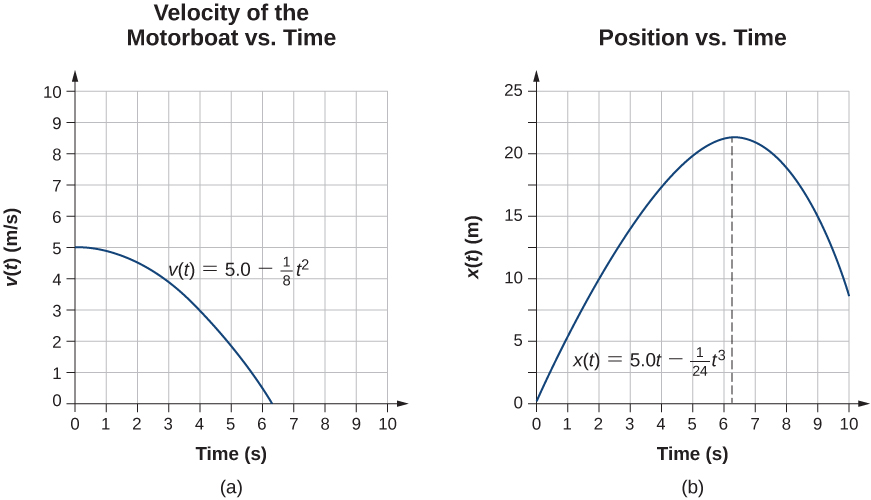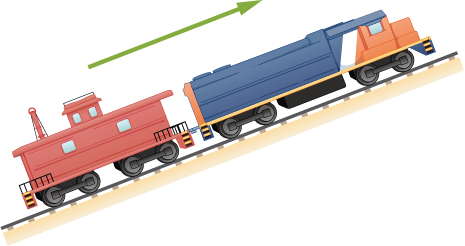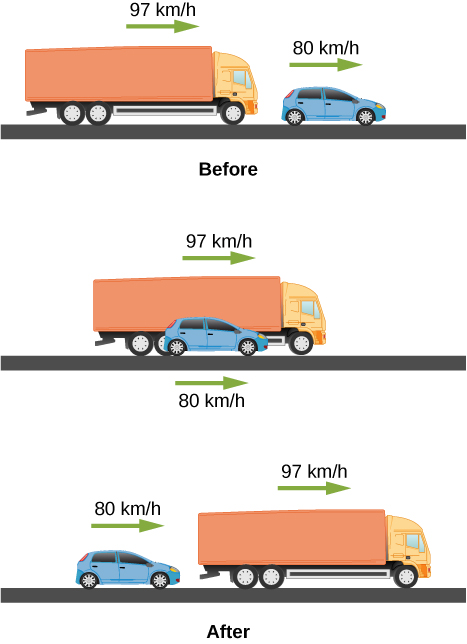## 3.6 Finding Velocity and Displacement from Acceleration

### Learning Objectives

By the end of this section, you will be able to:

• Derive the kinematic equations for constant acceleration using integral calculus.
• Use the integral formulation of the kinematic equations in analyzing motion.
• Find the functional form of velocity versus time given the acceleration function.
• Find the functional form of position versus time given the velocity function.

This section assumes you have enough background in calculus to be familiar with integration. In Instantaneous Velocity and Speed and Average and Instantaneous Acceleration we introduced the kinematic functions of velocity and acceleration using the derivative. By taking the derivative of the position function we found the velocity function, and likewise by taking the derivative of the velocity function we found the acceleration function. Using integral calculus, we can work backward and calculate the velocity function from the acceleration function, and the position function from the velocity function.

### Kinematic Equations from Integral Calculus

Let’s begin with a particle with an acceleration a(t) is a known function of time. Since the time derivative of the velocity function is acceleration,

$\frac{d}{dt}v(t)=a(t),$

we can take the indefinite integral of both sides, finding

$\int \frac{d}{dt}v(t)dt=\int a(t)dt+{C}_{1},$

where C1 is a constant of integration. Since $\int \frac{d}{dt}v(t)dt=v(t)$, the velocity is given by

$v(t)=\int a(t)dt+{C}_{1}.$

Similarly, the time derivative of the position function is the velocity function,

$\frac{d}{dt}x(t)=v(t).$

Thus, we can use the same mathematical manipulations we just used and find

$x(t)=\int v(t)dt+{C}_{2},$

where C2 is a second constant of integration.

We can derive the kinematic equations for a constant acceleration using these integrals. With a(t) = a a constant, and doing the integration in (Figure), we find

$v(t)=\int adt+{C}_{1}=at+{C}_{1}.$

If the initial velocity is v(0) = v0, then

${v}_{0}=0+{C}_{1}.$

Then, C1 = v0 and

$v(t)={v}_{0}+at,$

which is (Equation). Substituting this expression into (Figure) gives

$x(t)=\int ({v}_{0}+at)dt+{C}_{2}.$

Doing the integration, we find

$x(t)={v}_{0}t+\frac{1}{2}a{t}^{2}+{C}_{2}.$

If x(0) = x0, we have

${x}_{0}=0+0+{C}_{2};$

so, C2 = x0. Substituting back into the equation for x(t), we finally have

$x(t)={x}_{0}+{v}_{0}t+\frac{1}{2}a{t}^{2},$

which is (Equation).

### Example

#### Motion of a Motorboat

A motorboat is traveling at a constant velocity of 5.0 m/s when it starts to decelerate to arrive at the dock. Its acceleration is $a(t)=-\frac{1}{4}t\,\text{m/}{\text{s}}^{2}$. (a) What is the velocity function of the motorboat? (b) At what time does the velocity reach zero? (c) What is the position function of the motorboat? (d) What is the displacement of the motorboat from the time it begins to decelerate to when the velocity is zero? (e) Graph the velocity and position functions.

#### Strategy

(a) To get the velocity function we must integrate and use initial conditions to find the constant of integration. (b) We set the velocity function equal to zero and solve for t. (c) Similarly, we must integrate to find the position function and use initial conditions to find the constant of integration. (d) Since the initial position is taken to be zero, we only have to evaluate the position function at $t=0$.

#### Solution

We take t = 0 to be the time when the boat starts to decelerate.

1. From the functional form of the acceleration we can solve (Figure) to get v(t):

2. Solve (Figure):Figure 3.30 (a) Velocity of the motorboat as a function of time. The motorboat decreases its velocity to zero in 6.3 s. At times greater than this, velocity becomes negative—meaning, the boat is reversing direction. (b) Position of the motorboat as a function of time. At t = 6.3 s, the velocity is zero and the boat has stopped. At times greater than this, the velocity becomes negative—meaning, if the boat continues to move with the same acceleration, it reverses direction and heads back toward where it originated.

#### Significance

The acceleration function is linear in time so the integration involves simple polynomials. In (Figure), we see that if we extend the solution beyond the point when the velocity is zero, the velocity becomes negative and the boat reverses direction. This tells us that solutions can give us information outside our immediate interest and we should be careful when interpreting them.

A particle starts from rest and has an acceleration function $5-10t{\text{m/s}}^{2}$. (a) What is the velocity function? (b) What is the position function? (c) When is the velocity zero?

### Summary

• Integral calculus gives us a more complete formulation of kinematics.
• If acceleration a(t) is known, we can use integral calculus to derive expressions for velocity v(t) and position x(t).
• If acceleration is constant, the integral equations reduce to (Figure) and (Figure) for motion with constant acceleration.

### Key Equations

 Displacement $\text{Δ}x={x}_{\text{f}}-{x}_{\text{i}}$ Total displacement $\text{Δ}{x}_{\text{Total}}=\sum \text{Δ}{x}_{\text{i}}$ Average velocity $\overset{\text{–}}{v}=\frac{\text{Δ}x}{\text{Δ}t}=\frac{{x}_{2}-{x}_{1}}{{t}_{2}-{t}_{1}}$ Instantaneous velocity $v(t)=\frac{dx(t)}{dt}$ Average speed $\text{Average speed}=\overset{\text{–}}{s}=\frac{\text{Total distance}}{\text{Elapsed time}}$ Instantaneous speed $\text{Instantaneous speed}=|v(t)|$ Average acceleration $\overset{\text{–}}{a}=\frac{\text{Δ}v}{\text{Δ}t}=\frac{{v}_{f}-{v}_{0}}{{t}_{f}-{t}_{0}}$ Instantaneous acceleration $a(t)=\frac{dv(t)}{dt}$ Position from average velocity $x={x}_{0}+\overset{\text{–}}{v}t$ Average velocity $\overset{\text{–}}{v}=\frac{{v}_{0}+v}{2}$ Velocity from acceleration $v={v}_{0}+at\enspace(\text{constant}\,a\text{)}$ Position from velocity and acceleration $x={x}_{0}+{v}_{0}t+\frac{1}{2}a{t}^{2}\enspace(\text{constant}\,a\text{)}$ Velocity from distance ${v}^{2}={v}_{0}^{2}+2a(x-{x}_{0})\enspace(\text{constant}\,a\text{)}$ Velocity of free fall $v={v}_{0}-gt\,\text{(positive upward)}$ Height of free fall $y={y}_{0}+{v}_{0}t-\frac{1}{2}g{t}^{2}$ Velocity of free fall from height ${v}^{2}={v}_{0}^{2}-2g(y-{y}_{0})$ Velocity from acceleration $v(t)=\int a(t)dt+{C}_{1}$ Position from velocity $x(t)=\int v(t)dt+{C}_{2}$

### Conceptual Questions

When given the acceleration function, what additional information is needed to find the velocity function and position function?

### Problems

The acceleration of a particle varies with time according to the equation $a(t)=p{t}^{2}-q{t}^{3}$. Initially, the velocity and position are zero. (a) What is the velocity as a function of time? (b) What is the position as a function of time?

Between t = 0 and t = t0, a rocket moves straight upward with an acceleration given by $a(t)=A-B{t}^{1\,\text{/}2}$, where A and B are constants. (a) If x is in meters and t is in seconds, what are the units of A and B? (b) If the rocket starts from rest, how does the velocity vary between t = 0 and t = t0? (c) If its initial position is zero, what is the rocket’s position as a function of time during this same time interval?

The velocity of a particle moving along the x-axis varies with time according to $v(t)=A+B{t}^{-1}$, where A = 2 m/s, B = 0.25 m, and $1.0\,\text{s}\le t\le 8.0\,\text{s}$. Determine the acceleration and position of the particle at t = 2.0 s and t = 5.0 s. Assume that $x(t=1\,\text{s})=0$.

A particle at rest leaves the origin with its velocity increasing with time according to v(t) = 3.2t m/s. At 5.0 s, the particle’s velocity starts decreasing according to [16.0 – 1.5(t – 5.0)] m/s. This decrease continues until t = 11.0 s, after which the particle’s velocity remains constant at 7.0 m/s. (a) What is the acceleration of the particle as a function of time? (b) What is the position of the particle at t = 2.0 s, t = 7.0 s, and t = 12.0 s?

Professional baseball player Nolan Ryan could pitch a baseball at approximately 160.0 km/h. At that average velocity, how long did it take a ball thrown by Ryan to reach home plate, which is 18.4 m from the pitcher’s mound? Compare this with the average reaction time of a human to a visual stimulus, which is 0.25 s.

An airplane leaves Chicago and makes the 3000-km trip to Los Angeles in 5.0 h. A second plane leaves Chicago one-half hour later and arrives in Los Angeles at the same time. Compare the average velocities of the two planes. Ignore the curvature of Earth and the difference in altitude between the two cities.

Unreasonable Results A cyclist rides 16.0 km east, then 8.0 km west, then 8.0 km east, then 32.0 km west, and finally 11.2 km east. If his average velocity is 24 km/h, how long did it take him to complete the trip? Is this a reasonable time?

An object has an acceleration of $+1.2\,{\text{cm/s}}^{2}$. At $t=4.0\,\text{s}$, its velocity is $-3.4\,\text{cm/s}$. Determine the object’s velocities at $t=1.0\,\text{s}$ and $t=6.0\,\text{s}$.

A particle moves along the x-axis according to the equation $x(t)=2.0-4.0{t}^{2}$ m. What are the velocity and acceleration at $t=2.0$ s and $t=5.0$ s?

A particle moving at constant acceleration has velocities of $2.0\,\text{m/s}$ at $t=2.0$ s and $-7.6\,\text{m/s}$ at $t=5.2$ s. What is the acceleration of the particle?

A train is moving up a steep grade at constant velocity (see following figure) when its caboose breaks loose and starts rolling freely along the track. After 5.0 s, the caboose is 30 m behind the train. What is the acceleration of the caboose?An electron is moving in a straight line with a velocity of $4.0\,×\,{10}^{5}$ m/s. It enters a region 5.0 cm long where it undergoes an acceleration of $6.0\,×\,{10}^{12}\,{\text{m/s}}^{2}$ along the same straight line. (a) What is the electron’s velocity when it emerges from this region? b) How long does the electron take to cross the region?

An ambulance driver is rushing a patient to the hospital. While traveling at 72 km/h, she notices the traffic light at the upcoming intersections has turned amber. To reach the intersection before the light turns red, she must travel 50 m in 2.0 s. (a) What minimum acceleration must the ambulance have to reach the intersection before the light turns red? (b) What is the speed of the ambulance when it reaches the intersection?

A motorcycle that is slowing down uniformly covers 2.0 successive km in 80 s and 120 s, respectively. Calculate (a) the acceleration of the motorcycle and (b) its velocity at the beginning and end of the 2-km trip.

A cyclist travels from point A to point B in 10 min. During the first 2.0 min of her trip, she maintains a uniform acceleration of $0.090\,{\text{m/s}}^{2}$. She then travels at constant velocity for the next 5.0 min. Next, she decelerates at a constant rate so that she comes to a rest at point B 3.0 min later. (a) Sketch the velocity-versus-time graph for the trip. (b) What is the acceleration during the last 3 min? (c) How far does the cyclist travel?

Two trains are moving at 30 m/s in opposite directions on the same track. The engineers see simultaneously that they are on a collision course and apply the brakes when they are 1000 m apart. Assuming both trains have the same acceleration, what must this acceleration be if the trains are to stop just short of colliding?

A 10.0-m-long truck moving with a constant velocity of 97.0 km/h passes a 3.0-m-long car moving with a constant velocity of 80.0 km/h. How much time elapses between the moment the front of the truck is even with the back of the car and the moment the back of the truck is even with the front of the car?A police car waits in hiding slightly off the highway. A speeding car is spotted by the police car doing 40 m/s. At the instant the speeding car passes the police car, the police car accelerates from rest at 4 m/s2 to catch the speeding car. How long does it take the police car to catch the speeding car?

Pablo is running in a half marathon at a velocity of 3 m/s. Another runner, Jacob, is 50 meters behind Pablo with the same velocity. Jacob begins to accelerate at 0.05 m/s2. (a) How long does it take Jacob to catch Pablo? (b) What is the distance covered by Jacob? (c) What is the final velocity of Jacob?

Unreasonable results A runner approaches the finish line and is 75 m away; her average speed at this position is 8 m/s. She decelerates at this point at 0.5 m/s2. How long does it take her to cross the finish line from 75 m away? Is this reasonable?

An airplane accelerates at 5.0 m/s2 for 30.0 s. During this time, it covers a distance of 10.0 km. What are the initial and final velocities of the airplane?

Compare the distance traveled of an object that undergoes a change in velocity that is twice its initial velocity with an object that changes its velocity by four times its initial velocity over the same time period. The accelerations of both objects are constant.

An object is moving east with a constant velocity and is at position ${x}_{0}\,\text{at}\,\text{time}\,{t}_{0}=0$. (a) With what acceleration must the object have for its total displacement to be zero at a later time t ? (b) What is the physical interpretation of the solution in the case for $t\to \infty$?

A ball is thrown straight up. It passes a 2.00-m-high window 7.50 m off the ground on its path up and takes 1.30 s to go past the window. What was the ball’s initial velocity?

A coin is dropped from a hot-air balloon that is 300 m above the ground and rising at 10.0 m/s upward. For the coin, find (a) the maximum height reached, (b) its position and velocity 4.00 s after being released, and (c) the time before it hits the ground.

A soft tennis ball is dropped onto a hard floor from a height of 1.50 m and rebounds to a height of 1.10 m. (a) Calculate its velocity just before it strikes the floor. (b) Calculate its velocity just after it leaves the floor on its way back up. (c) Calculate its acceleration during contact with the floor if that contact lasts 3.50 ms $(3.50\,×\,{10}^{-3}\,\text{s})$ (d) How much did the ball compress during its collision with the floor, assuming the floor is absolutely rigid?

Unreasonable results. A raindrop falls from a cloud 100 m above the ground. Neglect air resistance. What is the speed of the raindrop when it hits the ground? Is this a reasonable number?

Compare the time in the air of a basketball player who jumps 1.0 m vertically off the floor with that of a player who jumps 0.3 m vertically.

Suppose that a person takes 0.5 s to react and move his hand to catch an object he has dropped. (a) How far does the object fall on Earth, where $g=9.8\,{\text{m/s}}^{2}?$ (b) How far does the object fall on the Moon, where the acceleration due to gravity is 1/6 of that on Earth?

A hot-air balloon rises from ground level at a constant velocity of 3.0 m/s. One minute after liftoff, a sandbag is dropped accidentally from the balloon. Calculate (a) the time it takes for the sandbag to reach the ground and (b) the velocity of the sandbag when it hits the ground.

(a) A world record was set for the men’s 100-m dash in the 2008 Olympic Games in Beijing by Usain Bolt of Jamaica. Bolt “coasted” across the finish line with a time of 9.69 s. If we assume that Bolt accelerated for 3.00 s to reach his maximum speed, and maintained that speed for the rest of the race, calculate his maximum speed and his acceleration. (b) During the same Olympics, Bolt also set the world record in the 200-m dash with a time of 19.30 s. Using the same assumptions as for the 100-m dash, what was his maximum speed for this race?

An object is dropped from a height of 75.0 m above ground level. (a) Determine the distance traveled during the first second. (b) Determine the final velocity at which the object hits the ground. (c) Determine the distance traveled during the last second of motion before hitting the ground.

A steel ball is dropped onto a hard floor from a height of 1.50 m and rebounds to a height of 1.45 m. (a) Calculate its velocity just before it strikes the floor. (b) Calculate its velocity just after it leaves the floor on its way back up. (c) Calculate its acceleration during contact with the floor if that contact lasts 0.0800 ms $(8.00\,×\,{10}^{-5}\,\text{s})$ (d) How much did the ball compress during its collision with the floor, assuming the floor is absolutely rigid?

An object is dropped from a roof of a building of height h. During the last second of its descent, it drops a distance h/3. Calculate the height of the building.

### Challenge Problems

In a 100-m race, the winner is timed at 11.2 s. The second-place finisher’s time is 11.6 s. How far is the second-place finisher behind the winner when she crosses the finish line? Assume the velocity of each runner is constant throughout the race.

The position of a particle moving along the x-axis varies with time according to $x(t)=5.0{t}^{2}-4.0{t}^{3}$ m. Find (a) the velocity and acceleration of the particle as functions of time, (b) the velocity and acceleration at t = 2.0 s, (c) the time at which the position is a maximum, (d) the time at which the velocity is zero, and (e) the maximum position.

A cyclist sprints at the end of a race to clinch a victory. She has an initial velocity of 11.5 m/s and accelerates at a rate of 0.500 m/s2 for 7.00 s. (a) What is her final velocity? (b) The cyclist continues at this velocity to the finish line. If she is 300 m from the finish line when she starts to accelerate, how much time did she save? (c) The second-place winner was 5.00 m ahead when the winner started to accelerate, but he was unable to accelerate, and traveled at 11.8 m/s until the finish line. What was the difference in finish time in seconds between the winner and runner-up? How far back was the runner-up when the winner crossed the finish line?

In 1967, New Zealander Burt Munro set the world record for an Indian motorcycle, on the Bonneville Salt Flats in Utah, of 295.38 km/h. The one-way course was 8.00 km long. Acceleration rates are often described by the time it takes to reach 96.0 km/h from rest. If this time was 4.00 s and Burt accelerated at this rate until he reached his maximum speed, how long did it take Burt to complete the course?